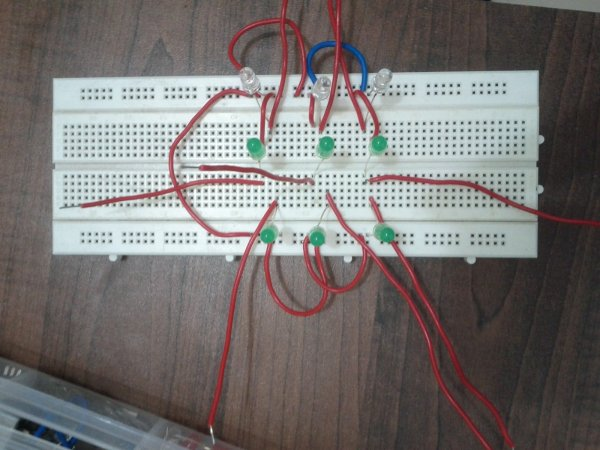# Arduino based Tic Tac Toe with TV RemoteThis was my first time attending the Internet of Things Pune Group. It was such a fun learning experience!

At today’s meetup, Dhiraj and Nishant started out by introducing the InduinoX and Arduino Uno boards along with the Processing IDE that is used to write the code.

After playing around with couple of sensors on the InduinoX we thought of doing a micro project together and finally settled on doing a Tic Tac Toe!

All those other ideas would hopefully make it into our next meetups!

### Following spec was decided for our micro-project.

1. A tic-tac-toe game with a mesh of 3×3 LEDs.
2. Controlled via a TV Remote. (IR). 1-9 keys on remote denote the position of the LED.
3. A player alternates using the TV Remote.
4. The first player’s LED is always bright (solids) and the second player’s LED blinks. (a novel idea considering we had only 1 color LED’s.)### Building It

1. We connected pins 2-10 of the Arduino Uno to a breadboard mounted with LED’s. Also the ground PIN was connected using jumpers. (as seen in the below pic.)
1. Code

2. view raw tic_tac_toe_arduino_ir.ino hosted withby GitHub
3. A video below shows the game play!
 #include int RECV_PIN = 11; IRrecv irrecv(RECV_PIN); decode_results results; int i = 0; int blinkarray; int user=0; void setup() { for(i=2;i<11;i++) { pinMode(i,OUTPUT); } irrecv.enableIRIn(); // Start the Remote receiver Serial.begin(9600); } void loop() { blinkOrNot(); if (irrecv.decode(&results)) { Serial.println(results.value); switch(results.value) // if the ‘+’ button is pressed { case 2320: i=0; break;// 2320 is the value for ‘0’ case 16: i=1; setArray(); break;// 16 is the value for ‘1’ case 2064: i=2; setArray(); break;// 2064 is the value for ‘2’ case 1040: i=3; setArray(); break;// 1040 is the value for ‘3’ case 3088: i=4; setArray(); break;// 3088 is the value for ‘4’ case 528: i=5; setArray(); break;// 528 is the value for ‘5’ case 2576: i=6; setArray(); break;// 2576 is the value for ‘6’ case 1552: i=7; setArray(); break;// 1552 is the value for ‘7’ case 3600: i=8; setArray(); break;// 1552 is the value for ‘7’ case 272: i=9; setArray(); break;// 1552 is the value for ‘7’ } // while(digitalRead(7)==0); // wait till button is released to avoid incrementing the counter again delay(300); // small delay to avoid debounce irrecv.resume(); // Receive the next value } }void blinkOrNot(){ for(int j=2;j<11;j++) { if(blinkarray[j]==1) { digitalWrite(j,HIGH); } } for(int j=2;j<11;j++){ if(blinkarray[j]==2) { digitalWrite(j,HIGH); } } for(int j=2;j<11;j++){ if(blinkarray[j]==2) { delay(50); } } for(int j=2;j<11;j++){ if(blinkarray[j]==2) { digitalWrite(j,LOW); } } for(int j=2;j<11;j++){ if(blinkarray[j]==2) { delay(50); } } }

Read more: Arduino based Tic Tac Toe with TV Remote